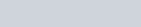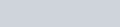Make correct statements by filling in the symbols ⊂ or ⊄ in the blank spaces:
Question:

Make correct statements by filling in the symbols ⊂ or ⊄ in the blank spaces:

(i) {2, 3, 4} … {1, 2, 3, 4, 5}

(ii) {abc} … {bcd}

(iii) {xx is a student of Class XI of your school} … {xx student of your school}

(iv) {xx is a circle in the plane} … {xx is a circle in the same plane with radius 1 unit}

(v) {xx is a triangle in a plane}…{xx is a rectangle in the plane}

(vi) {xx is an equilateral triangle in a plane}… {xx is a triangle in the same plane}

(vii) {xx is an even natural number} … {xx is an integer}

Solution:

(i)(ii)(iii) {xx is a student of class XI of your school}⊂ {xx is student of your school}

(iv) {xx is a circle in the plane} ⊄ {xx is a circle in the same plane with radius 1 unit}

(v) {xx is a triangle in a plane} ⊄ {xx is a rectangle in the plane}

(vi) {xx is an equilateral triangle in a plane}⊂ {xx in a triangle in the same plane}

(vii) {xx is an even natural number} ⊂ {xx is an integer}